### Statistics Fundamentals

In probability, we solve forwards. It starts with an exact and well-defined rule. We use this rule to predict what will happen in the future. We don't know the results, but probability allows us to quantify what should happen.

In statistics, we solve backwards. It starts with the end results, known as data. We take this data and try to determine what well-defined rules created it.

Let's take a look at some examples to better understand the difference.

# Solving Forwards, Solving Backwards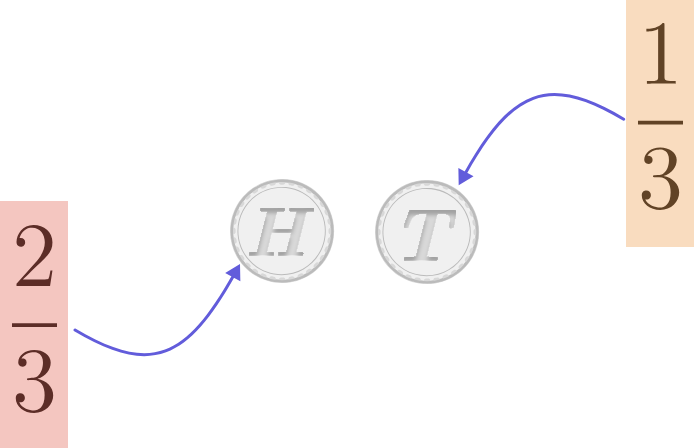Maja has an unfair coin which is weighted so that, when flipped, it has a $\frac{2}{3}$ chance of landing on heads and $\frac{1}{3}$ chance of landing on tails.

If she flips the coin twice in a row, what is the probability it shows the same side both times?

Take a look at the Probability Refresher quiz if this material seems unfamiliar.

# Solving Forwards, Solving Backwards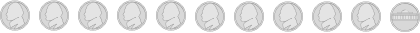Maja has a second coin which she thinks is unfair, just like her other coin. But she isn't sure. So she'd like to test the coin out by flipping it multiple times.

How many times does she need to flip the coin to be 100% certain that the coin is unfair?

# Solving Forwards, Solving Backwards

The first scenario was an example of probability. We knew the coin was unfair, with a $\frac{2}{3}$ chance of heads and a $\frac{1}{3}$ chance of tails on every flip. We used that rule to figure out the chance that the same side would come up for two flips in a row.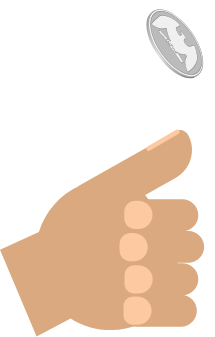The second scenario was an example of statistics. We wanted to find out an unknown: whether or not a coin was unfair. We need data to investigate this question. And using that data, we can figure out the likelihood that the coin is unfair given our results.

# Solving Forwards, Solving Backwards

Any statistical prediction should have some margin of error. That's because we are using data to estimate some unknown value.

For example, let's say we want to determine the number of fish in all of the lakes in the United States of America. To answer that question exactly, we would need to physically count all of the fish in every single lake. This is an impossible task. So we take a random smaller sample and use statistics to come up with an estimate and a margin of error.If our data leads us to make an estimate that the true number of fish in every lake in the United States is 9 billion $\pm$ 0.7 billion, what does that mean?

# Solving Forwards, Solving Backwards

Note in the previous problem we still have uncertainty as to our maximum and minimum. If we insisted on a range that covered 100% of possible errors, the range is potentially unlimited. So we pick some threshold of error we are willing to tolerate.

One tool for setting the threshold is assuming multiple samples would form a normal curve (or bell curve), as shown below. The height of the curve at a particular point indicates proportionally how many observations will fall there.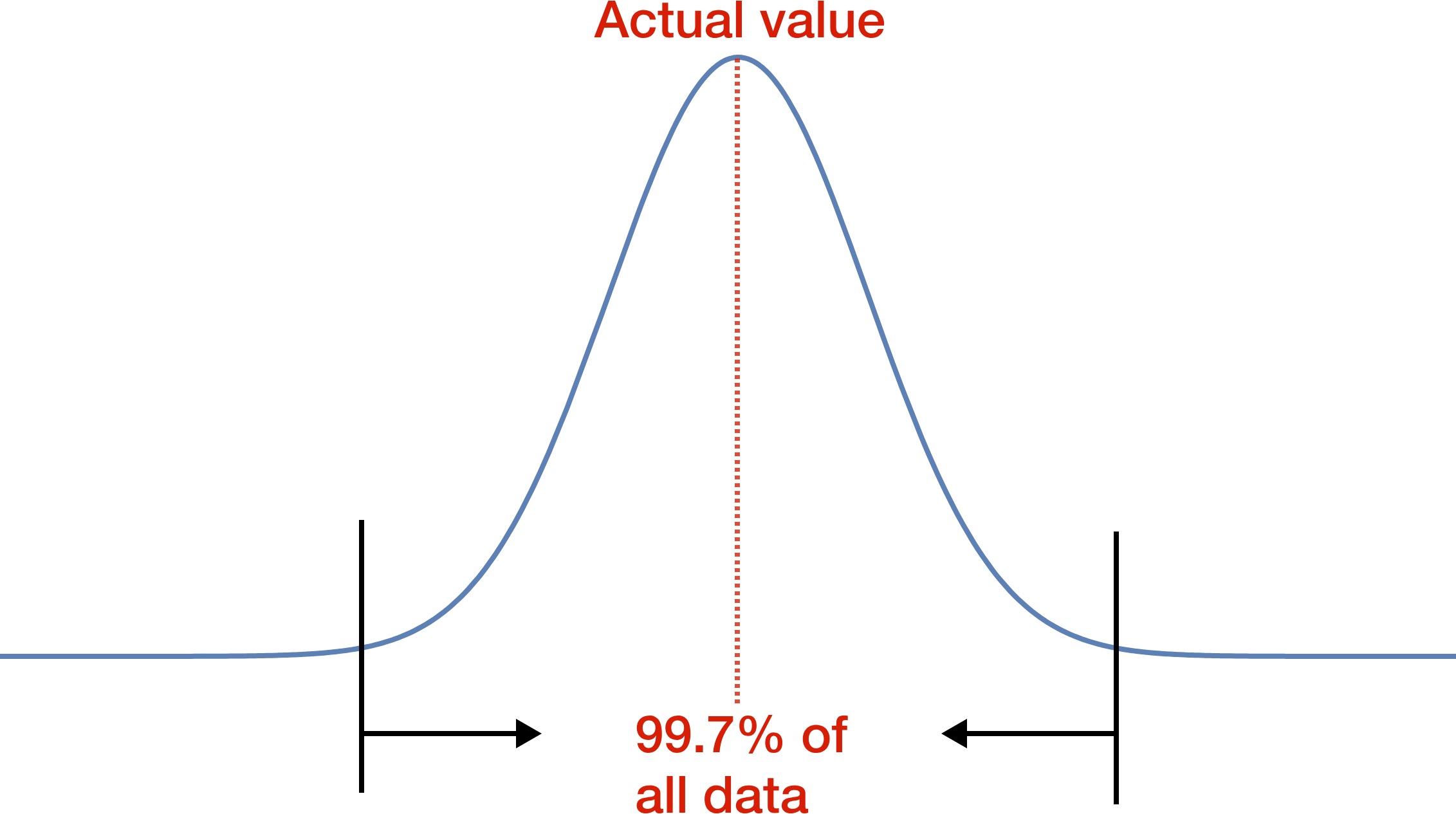This distribution is extremely popular in statistics because it applies to many real-world situations. As you can see, the most common observation is in the center (the mean). The majority of observations are close to the middle. And as we move to more extreme values on either side, the likelihood is less and less.

Which of the two curves below is marked correctly? (The 99.7% marks are left for clarity.)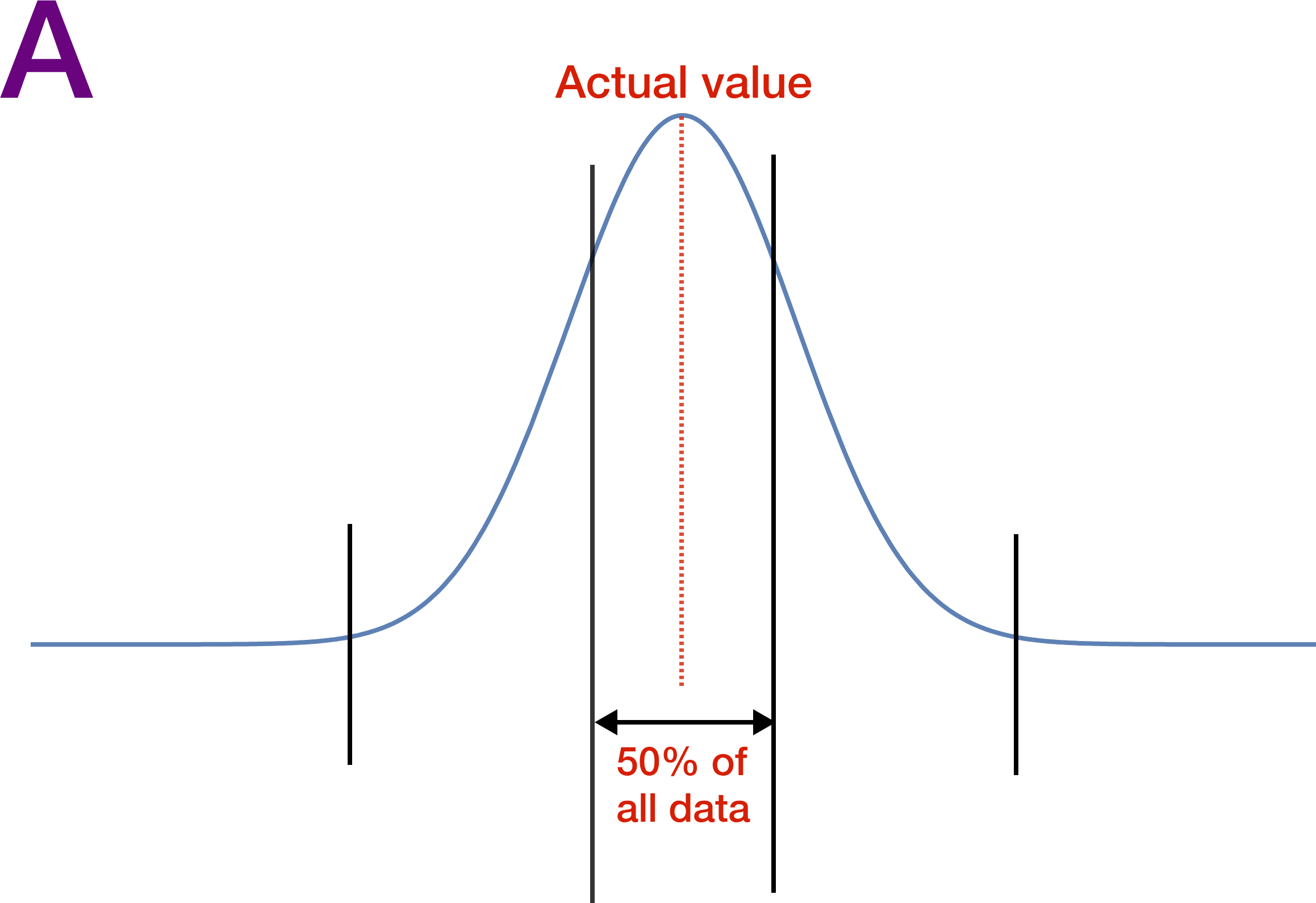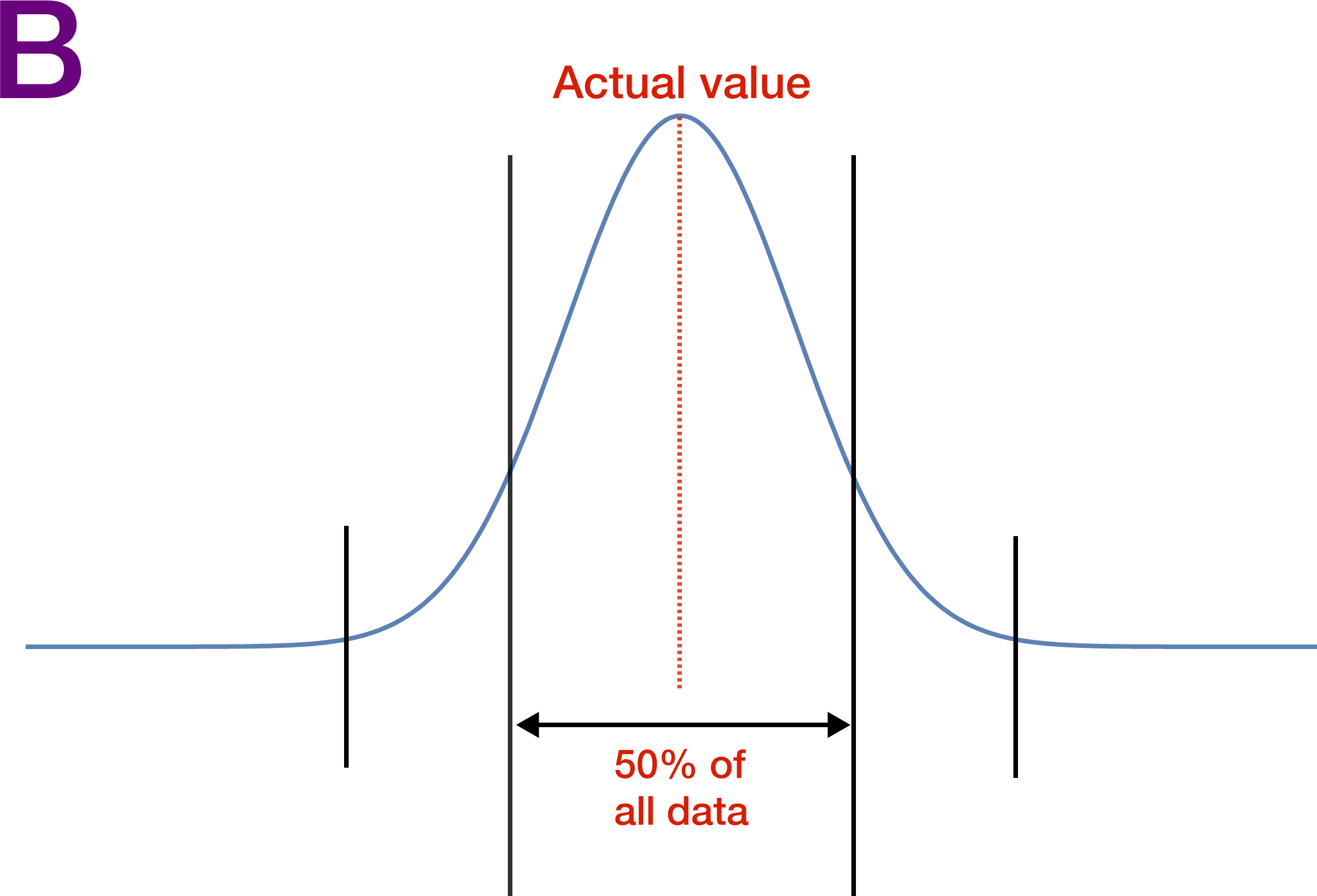# Solving Forwards, Solving Backwards

Let's step back and think on a smaller scale than the previous problem. Maja thinks her coin is weighted towards heads. She flips it 4 times and gets heads every time.She calculates that this would only occur with a fair coin roughly 6% of the time. So should she conclude there is a roughly 94% chance that her coin is weighted towards heads?

# Solving Forwards, Solving Backwards

The last problem showed how we must place a "threshold" on when we start to think data looks suspicious, but it is dependent on both judgment and context. And this threshold is not the same as the probability our conclusion is correct. We will examine this later in course!

Statistics requires us to solve backwards. We want to uncover real-world truths by analyzing what has already happened (i.e. data). But this quiz showed how we have to make many decisions along the way about how to analyze our data and solve backwards.

As a result, statistics can sometimes be misleading. Move on to the next quiz to explore some ways statistics can deceive us, both intentionally and unintentionally.

×